# Relativistic Collisions

© 2017-2018 Kevan Hashemi, Brandeis University

## Contents

Introduction
Failure of Classical Momentum
Relativistic Momentum
Relativistic Mass
Force and Acceleration
Kinetic Energy
Mass-Energy
Photons
Questions

## Introduction

This investigation of collisions between hypothetical fast-moving objects follows our Derivation of the Lorentz Transform. We will use γ to denote the Lorentz Factor at velocity v. We have:

γ = 1/√(1 − v2/c2),

where c is the speed of light. While the derivation of the Lorentz Transorm requires only simple algebra, the derivation of the equation for the kinetic energy of a fast-moving object requires an integral with change of variables. Even if you cannot follow the details of each derivation, we hope you will still be able to follow the text and understand the conclusions.

## Failure of Classical Momentum

Suppose we have two objects A and B. Object A is stationary in F, at position x = 0 m. To observers in F, object A has mass m. Object B is stationary in F | at x | = 0 m. To observers in F |, object B has mass m also.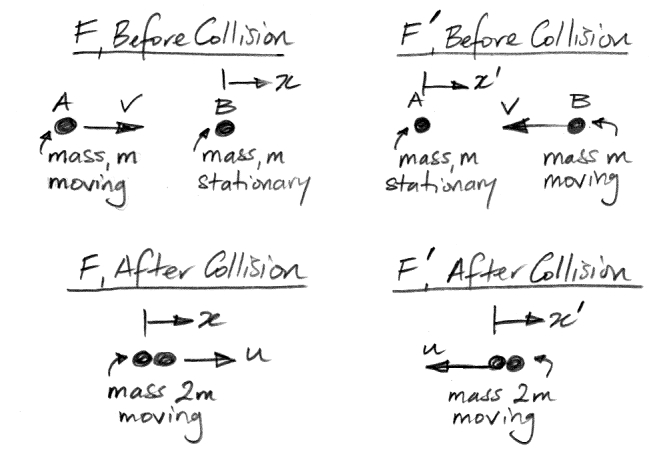Figure: Two Objects of Equal Mass Collide and Stick Together. One is initially stationary in F, the other in F |.

At time t = t | = 0 s, A and B collide and stick together at position x = x | = 0 m. In F, the combined A and B move off together at a velocity u in the x-direction. The symmetry of this arrangement, combined with the principle of relativity, dictate that the combined A and B must move off together at a velocity −u in the x |-direction.

The value of u must be such that velocity +u in F appears as −u in F |. Let us use x to represent the position of the combined A and B in F. Then x = ut. And let us use x | to represent the position of the combined A and B in F |. Then x | = −ut |. Using x = ut in the Lorentz Transformation we get:

x | = γ(utvt)
t | = γ(tvut/c2)

Using x | = −ut | we get:

γ(utvt) = −uγ(tvut/c2)
uv = −u(1 − vu/c2)
vu2/c2 − 2u + v = 0

We note that if u << c we have u = v/2, which is what we expect from conservation of momentum at low velocities. Applying the quadratic formula, we get two solutions for the relativistic value of u, only one of which is consistent with our construction.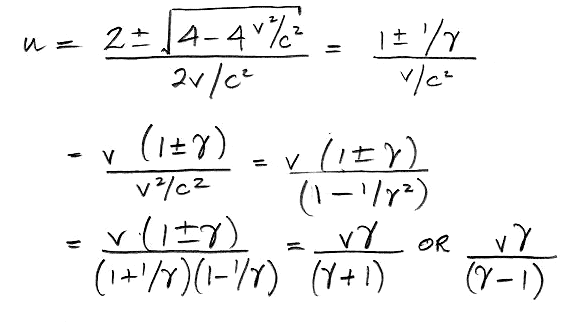We cannot have u > v, for that would mean that the combined A and B move off in F faster than object A struck B. But γ/(γ−1) >1, so the value of u is:

u = γv / (γ + 1) ≥ u/2

According to classical mechanics, for an observer in F, the momentum of A before the collision is mv, and that of B is zero. After the collision, A and B combined have momentum 2mu. Using the law of conservation of momentum, we conclude that u = v/2. At low velocities, γ ≈ 1, so we do indeed have u = v/2. But at higher velocities, u > v/2. Relativistic collisions do not obey the classical law of conservation of momentum.

According to classical mechanics, the kinetic energy of A before the collision, as calculated by an observer in F, is mv2/2. The kinetic energy of B before the collision is zero. After the collision, the kinetic energy of A and B combined is 2mu2/2. Suppose v = 0.999c and the masses of A and B are 1 kg. The initial kinetic energy is mv2/2 = 0.499c2. We have γ = 22, so our argument from symmetry dictates that u = 0.956c, irrespective of any argument from conservation of momentum, mass, or energy. After the collision, the kinetic energy of A and B combined is 2mu2/2 = 0.914c2, which is greater than the initial kinetic energy. Relativistic collisions do not obey the classical law of conservation of energy.

The principle of relativity and the uniformity of the speed of light supersedes any other law of conservation we might propose. They contradict the classical laws of motion. We need new laws of motion so that we can predict the outcome of relativistic collisions.

## Relativistic Momentum

If classical momentum and energy are not conserved in relativistic collisions, we must ask ourselves what it conserved, for otherwise we will be unable to predict the outcome of such collisions. Let us modify our previous collision example. Suppose that B has velocity δ << v in the y-direction, where y is perpendicular to x, as shown below.Figure: Symmetric, Inelastic Collision with Small Sideways Velocities.

In F |, we have the y | parallel to y. There is no movement of F | with respect to F in the y-direction. The observer in F sees a contraction of x | and t |. Does she see a contraction in y |? Suppose she shines a ray of light in the y-direction at t = x = 0. At time t = 1 s, the ray of light arrives at x = 0 m and y = c × 1 s. Applying the Lorentz Transform, the ray of light has arrived at x | = −γv × 1 s at time t | = γ × 1 s, and at some value of y | that we have yet to determine. But we do know that the observer in F | must see the ray of light propagating at velocity c, and this knowledge allows us to determine y |.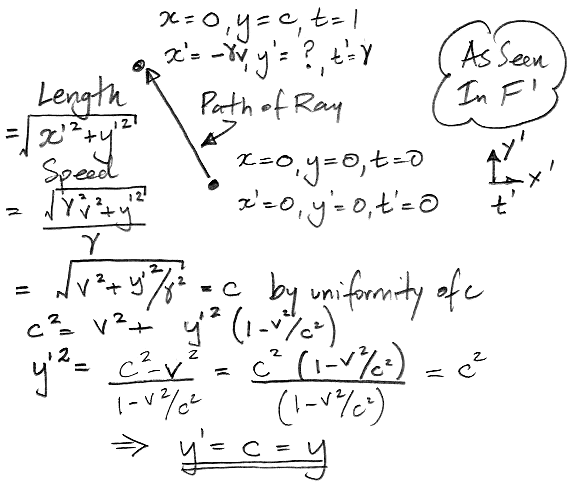Figure: A Sideways Light Ray Must Move at c For All Observers.

There is no contraction of length in the y-direction. By the same argument, we can show that there will be no contraction of length in any direction perpendicular to the x-direction.

Let us return to B moving at small velocity δ in the y-direction. Suppose B is at y = y | = 0 m at time t = t | = 0 s. At time t = 1 s, B is at y = δ × 1 s, which is coincident with t | = γ × 1 s and y | = δ × 1 s. In F |, the sideways velocity of B is δ/γ, which is smaller than δ.

Suppose we give A a velocity −δ in the y |-direction as observed in F |. This sideways velocity will appear to be −δ/γ in F. Suppose A and B collide and stick together. By symmetry, the velocity of the combined object in the y-direction must be zero. To observers in both frames, one object of mass m and y-speed −δ/γ collides with another of the same mass but opposite y-speed δ and the result is no movement in y at all.

Because δ << v, δ has no significant effect upon the speed of A as seen in F, nor upon the speed of B as seen in F |, and is so much smaller than c that 1/√(1−δ2/c2) is almost exactly equal to one. In F, object A behaves as if its mass were γm, so that its momentum in the y-direction is −γmδ/γ = −δm, exactly opposite to the momentum of B.

We invite you to construct further, symmetric collision examples for yourself. You will find that an object moving at velocity w in frame F acts as if it has mass mγ, where γ = 1/√(1−w2/c2) and m is the object's rest mass or invariant mass, which is its mass measured in a frame in which the object is stationary. We call γm the relativistic mass of A in F due to its velocity w. An object's relativistic momentum is its relativistic mass multiplied by its velocity. Classical momentum is not conserved in relativistic collisions, but relativistic momentum is.

Law: The sum of relativistic momentum before a collision is equal to the sum of relativistic momentum after the collision.

In contemporary physics literature, momentum means γmv. When we write only mv for momentum, that's because we are assumeing γ ≈ 1.

## Relativistic Mass

Let us return to our inelastic collision between two objects, one stationary in F and the other stationary in F |. Let us use conservation of rest mass and relativistic momentum to deduce the final velocity of the combined object, and see if we get the same answer as we did by application of the principle of relativity alone.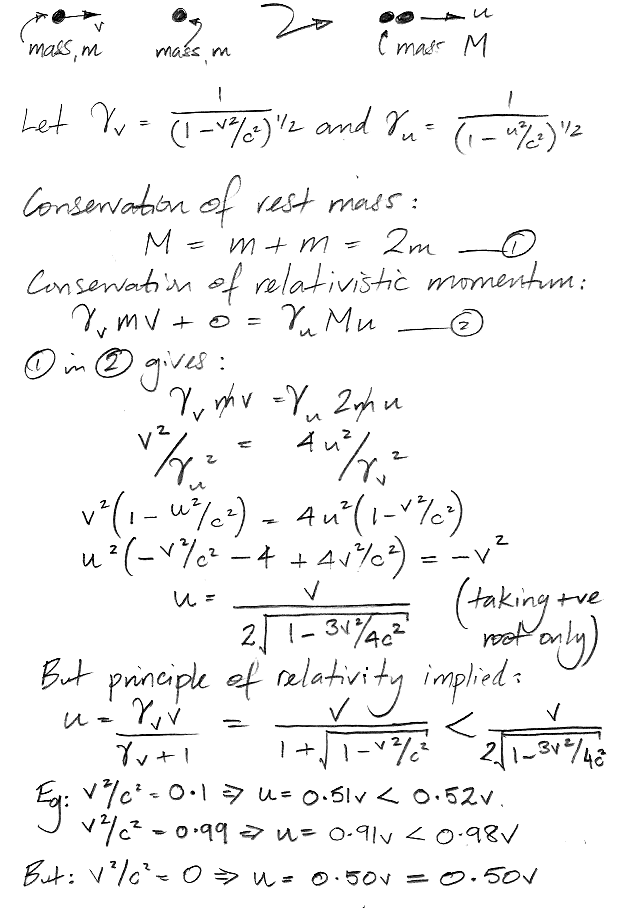Figure: An Attempt To Calculate Final Velocity By Assuming Conservation of Rest Mass and Relativistic Momentum.

We don't get the same answer: our final velocity is too high. Either this collision fails to conserve relativistic momentum or it fails to conserve rest mass. Following our sideways-velocity example in the section above, it's hard to see how relativistic collisions can be consistent with the principle of relativity if they do not conserve relativistic momentum. But we have no reason to assume that conservation of rest mass should apply to relativistic collisions. Let's see how the calculation works out if we assume conservation of relativistic mass instead of conservation of rest mass.Figure: A Collision Analyzed Using Conservation of Relativistic Mass and Momentum.

Conservation of relativistic mass and momentum lead us to the same value of u as the Lorentz Transformation and the principle of relativity. And they give us something more: the combined rest mass of the two objects after the collision. When γv = 1 this mass is 2m, as we would expect from the classical law of conservation of mass. But when γ = 10, the combined rest mass after the collision is 4.7m.

Law: The sum of relativistic masses before a collision is equal to the sum of relativistic masses after the collision.

With these two conservation laws that we can predict accurately the outcome of any collision, in particular the outcome of collisions between sub-atomic particles moving close to the speed of light.

## Force and Acceleration

We can express Newton's Second Law as "Force equals mass times acceleration" or "Force equals the rate of change of momentum". Both definitions are equivalent at velocities much lower than the speed of light. But they are not equivalent for fast-moving objects. The following thought experiment proves that it is the rate of change of momentum statement that is universal.

Suppose we have two soft, inelastic rods moving parallel to their own lengths so as to collide with one another. We also have two rigid plates held apart by a stiff spring. The plates are held above the ground by a pair of leaf springs so that the plates interpose themselves between the two rods. At time t = 0, Rod 1 strikes the first plate and Rod 2 strikes the second plate.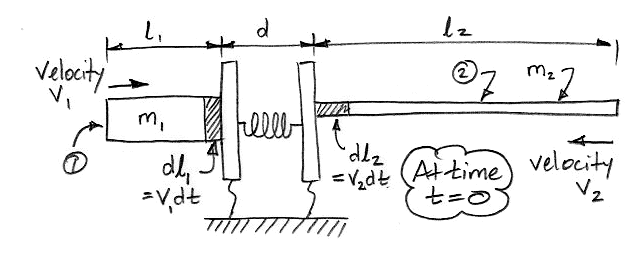Figure: Simultaneous Collision of Two Soft, Inelastic Rods with Force-Measuring Plates.

The rods are soft and inelastic. Whatever material strikes the plates comes to an immediate stop in the direction perpendicular to the plate surface, and spreads out parallel to the plate surface. The momentum of the material stopping on the plates compresses the spring with force F (apologies for using F to denote a force and to name a frame of reference). We assume the spring is stiff, so the change in the plate separation is small. We choose the velocity, mass, and length of the two rods so that the force exerted by the first rod is equal to the force exerted by the second, and also so that the two rods finish colliding with the plates at the same time t = T.

At time T the material of the two rods has no momentum in the direction perpendicular to the plates. By the law of conservation of relativistic momentum, we see that the initial momentum of the first rod must have been equal to the initial momentum of the second rod.

p1 = γ1m1v1 = p2 = γ2m2v2

Here p denotes momentum towards the collision, and γ the Lorentz Factor, for each object with respect to the ground. Consider the two elements colliding with the plates during an interval of time dt. They have momentum dp1 and dp2 respectively. The mass of these elements is in proportion to their length, which we will observe from the frame of reference of the ground.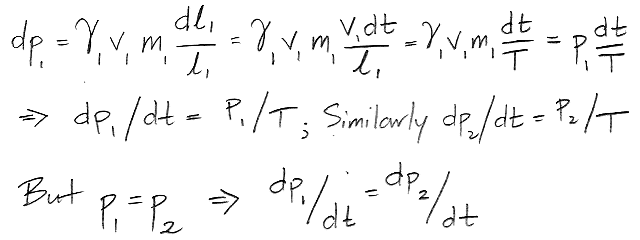Now suppose that v1 << c so that γ1 ≈ 1. We know from Newton's Second Law that the force F exerted by the collision of Rod 1 with the left-hand plate will be equal to dp1/dt, which is in turn equal to dp2/dt. By construction, however, this force is the same as the one exerted by Rod 2 on the right-hand plate, so we also have F = dp2/dt. The force exerted by either rod is equal to the rate of change of its relativistic momentum, regardless of how fast it is moving.

Law: The force upon an object is F = dp/dt, where p= γmv is the relativistic momentum.

This is Newton's Second Law for all velocities. For γ ≈ 1, the momentum is roughly equal to mv and we can use the approximation F = ma, where a is the acceleration.

## Kinetic Energy

Suppose an observer in frame F sees a force F accelerate an object of rest mass m from velocity zero to v. How much work does this force do? This work is what we call the moving object's kinetic energy. In the following derivation we calculate the kinetic energy using our equation for force in terms of the rate of change of relativistic momentum.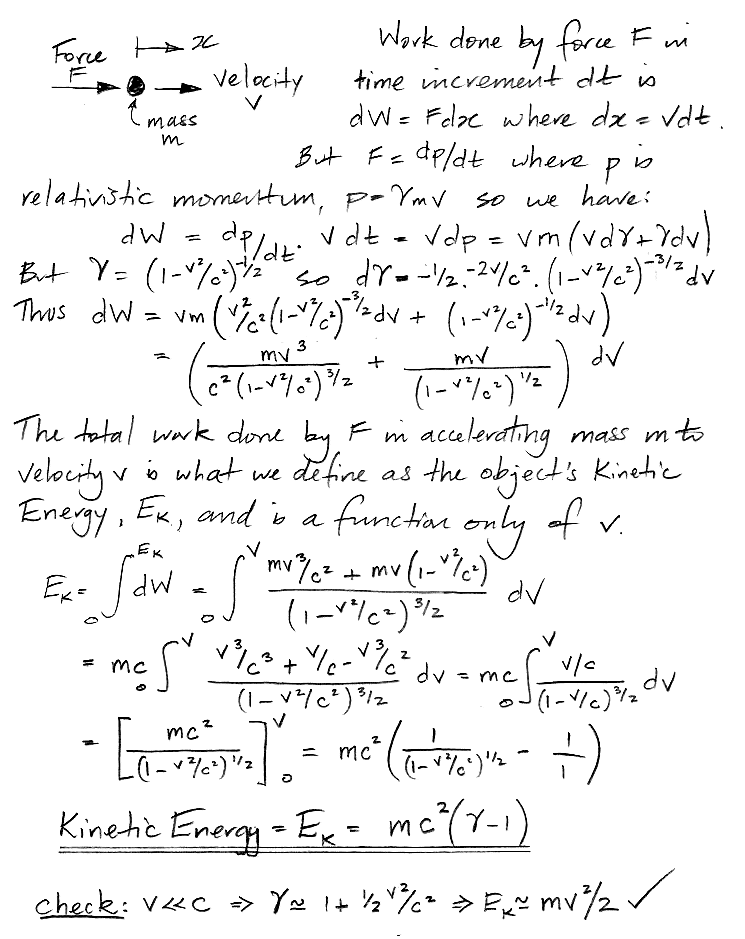The kinetic energy of an object is mv(γ − 1), and this equation applies equally well at all velocities.

Coming soon...

Coming soon...

## Questions

Here are some exercises.

1. A lump of metal weighing 1 g and at temperature 20°C is traveling at 99.9% the speed of light. It collides with a stationary lump of metal weighing 1 kg and at 20°C. The 1-g lump enters the larger lump and is absorbed and slowed down by material friction alone. The two move off together and at the same speed. How fast does the combined lump move? What is the mass of the combined lump? The lump radiates heat into space until its temperature drops to 20°C. What is its mass now? How much heat did it radiate?